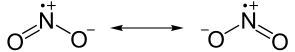## Formal Charge and Lewis Structure

#### Learning Objective

• Calculate formal charges on atoms in a compound

#### Key Points

• Lewis structures, also called Lewis dot diagrams, model covalent bonding between atoms. These diagrams use dots around atoms to signify electrons and lines to signify bonds between atoms.
• Lewis structures incorporate an atom’s formal charge, which is the charge on an atom in a molecule, assuming that electrons in a chemical bond are shared equally between atoms.
• When multiple Lewis structures can represent the same compound, the different Lewis formulas are called resonance structures.

#### Terms

• resonance structureA molecule or polyatomic ion that has multiple Lewis structures because bonding can be shown multiple ways.
• octet ruleAtoms lose, gain, or share electrons in order to have a full valence shell of eight electrons.
• formal chargeThe charge assigned to an atom in a molecule, assuming that electrons in a chemical bond are shared equally between atoms. This helps determine which of a few Lewis structures is most correct.

## Lewis Structures

Pictorial representations are often used to visualize electrons, as well as any bonding that may occur between atoms in a molecule. In particular, chemists use Lewis structures (also known as Lewis dot diagrams, electron dot diagrams, or electron structures) to represent covalent compounds. In these diagrams, valence electrons are shown as dots that sit around the atom; any bonds that the atoms share are represented by single, double, or triple lines.The Lewis dot structure of carbonA typical Lewis structure of carbon, with the valence electrons denoted as dots around the atom.

Generally, most Lewis structures follow the octet rule; they will share electrons until they achieve 8 electrons in their outermost valence shell. However, there are exceptions to the octet rule, such as boron, which is stable with only 6 electrons in its valence shell. The elements hydrogen (H) and helium (He) follow the duet rule, which says their outermost valence shell is full with 2 electrons in it.

## Drawing a Lewis Structure

To draw a Lewis structure, the number of valence electrons on each atom in the compound must be determined. The total number of valence electrons in the entire compound is equal to the sum of the valence electrons of each atom in the compound. Non-valence electrons are not represented when drawing the Lewis structures.

Valence electrons are placed as lone pairs (two electrons) around each atom. Most atoms may have an incomplete octet of electrons. However, atoms can share electrons with each other to fulfill this octet requirement. A bond that shares two electrons is called a single bond and is signified by a straight, horizontal line.

If the octet rule is still not satisfied, atoms may form a double (4 shared electrons) or triple bond (6 shared electrons). Because the bonding pair is shared, the atom that had the lone pair still has an octet, and the other atom gains two or more electrons in its valence shell.

For example, CO2 is a neutral molecule with 16 total valence electrons. In the Lewis structure, carbon should be double-bonded to both oxygen atoms.The Lewis structure for carbon dioxideThis diagram shows the conceptual stages of drawing the Lewis structure for a molecule of carbon dioxide (CO2).

Lewis structures can also be drawn for ions. In these cases, the entire structure is placed in brackets, and the charge is written as a superscript on the upper right, outside of the bracket.The Lewis structure for the hydroxide ionIn the hydroxide ion (OH–), the entire structure is surrounded by a bracket, and the charge is placed outside the bracket.

## Determining Formal Charge

Although we know how many valence electrons are present in a compound, it is harder to determine around which atoms the electrons actually reside. To assist with this problem, chemists often calculate the formal charge of each atom. The formal charge is the electric charge an atom would have if all the electrons were shared equally.

The formal charge of an atom can be determined by the following formula:

$FC = V - (N + \frac{B}{2})$

In this formula, V represents the number of valence electrons of the atom in isolation, N is the number of non-bonding valence electrons, and B is the total number of electrons in covalent bonds with other atoms in the molecule.

For example, let’s calculate the formal charge on an oxygen atom in a carbon dioxide (CO2) molecule:

FC = 6 valence electrons – (4 non-bonding valence electrons + 4/2 electrons in covalent bonds)

FC = 6 – 6 = 0

The oxygen atom in carbon dioxide has a formal charge of 0.

## Resonance Structures

Sometimes multiple Lewis structures can be drawn to represent the same compound. These equivalent structures are known as resonance structures and involve the shifting of electrons and not of actual atoms. Depending on the compound, the shifting of electrons may cause a change in formal charges. Most often, Lewis structures are drawn so that the the formal charge of each atom is minimized.Resonance structuresTwo of the contributing structures of nitrogen dioxide (NO2). Both formulas represent the same number of atoms and electrons, just in a slightly different conformation.